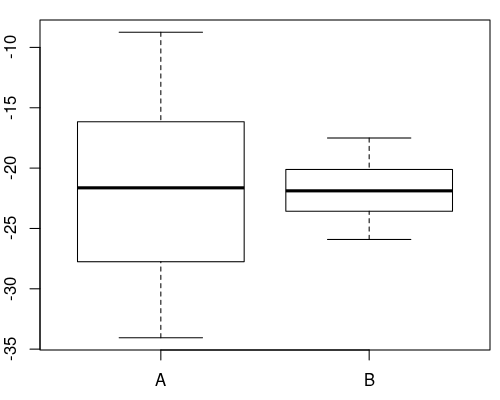## Exam 1

1. #### Question

In the following figure the distributions of a variable given by two samples (A and B) are represented by parallel boxplots. Which of the following statements are correct? (Comment: The statements are either about correct or clearly wrong.)1. The location of both distributions is about the same.
2. Both distributions contain no outliers.
3. The spread in sample A is clearly bigger than in B.
4. The skewness of both samples is similar.
5. Distribution A is right-skewed.

#### Solution

1. True. Both distributions have a similar location.
2. True. Both distributions have no observations which deviate more than 1.5 times the interquartile range from the box.
3. True. The interquartile range in sample A is clearly bigger than in B.
4. True. The skewness of both distributions is similar, both are about symmetric.
5. False. Distribution A is about symmetric.